# Percentage of Completion Method

The recognition of revenue on an ongoing basis depending on the stages of a project’s completion

## What is the Percentage of Completion Method?

The percentage of completion method of revenue recognition is a concept in accounting that refers to a method by which a business recognizes revenue on an ongoing basis depending on the stages of a project’s completion. In other words, the percentage of completion method is used for longer-term projects and recognizes revenue and expenses as a percentage of the project’s completion during the period.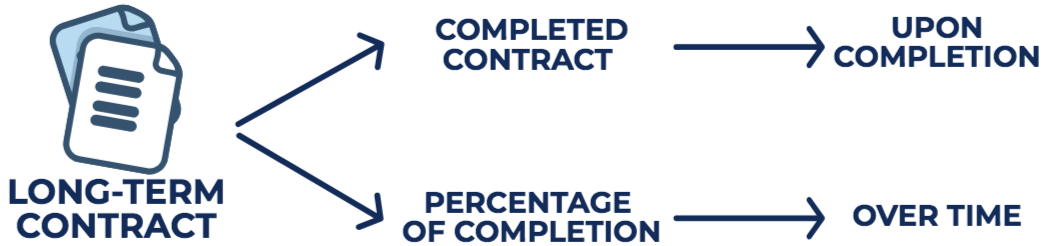### Understanding the Percentage of Completion Method

The percentage of completion method falls in-line with IFRS 15, which indicates that revenue from performance obligations recognized over a period of time should be based on the percentage of completion. The method recognizes revenues and expenses in proportion to the completeness of the contracted project. It is commonly measured through the cost-to-cost method.

There are two conditions to use the percentage of completion method:

1. Collections by the company must be reasonably assured.
2. Costs and project completion must be reasonably estimated.

### Journal Entries: Percentage of Completion Method

Journal entries for the percentage of completion method are as follows: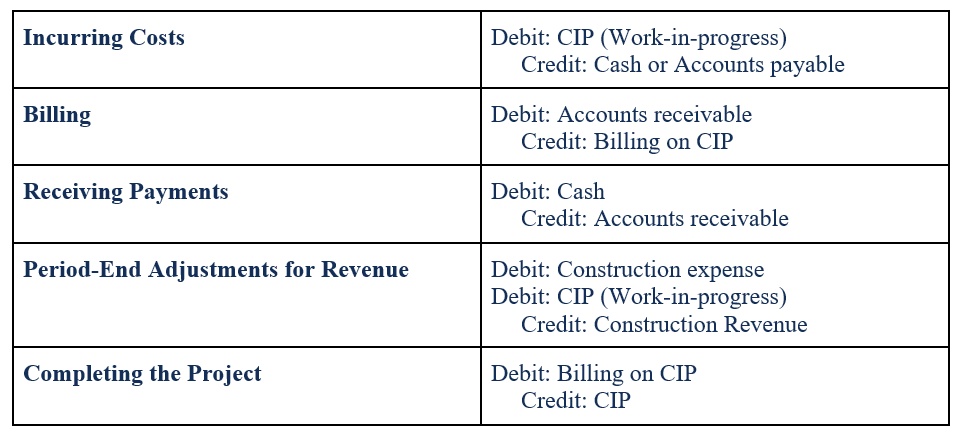### Cost-To-Cost Approach

In the cost-to-cost approach, the percentage of completion is based on the costs incurred to the estimated total cost to complete the project. Therefore, the equation for the cost-to-cost estimate of percentage completion is:

Percentage complete: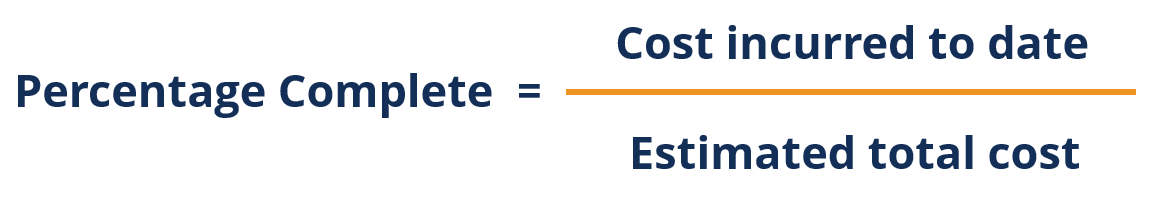Revenue recognized: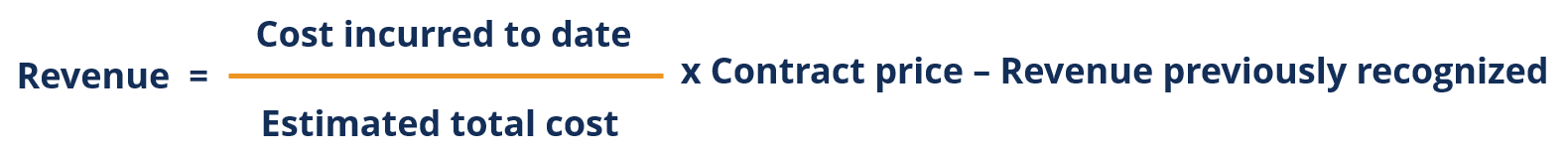An example is provided below to clarify the cost-to-cost approach.

### Example of the Cost-To-Cost Approach

StrongBridges Ltd. was awarded a \$20 million contract to build a bridge. The estimated time to complete the project is three (3) years, with an estimated cost of \$15 million. Assuming that the cost estimates do not change, the project is expected to generate \$5 million in profit. The following is a schedule on the project: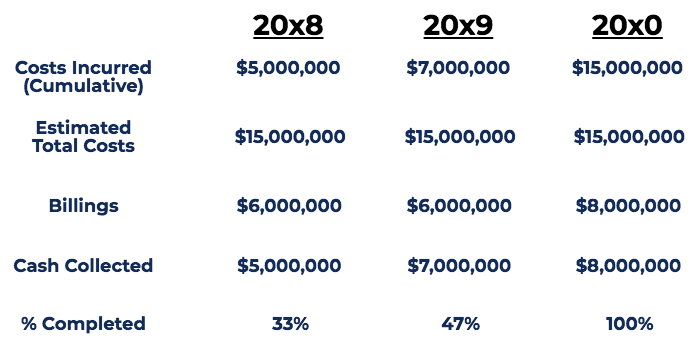Notes:

• Costs Incurred is the costs incurred to build the bridge as estimated by the company’s engineer.
• Billings are the amount of money StrongBridges Ltd. billed for the construction of the bridge. Billings amount is set by the company.
• Cash Collected is the amount of money StrongBridges Ltd. received for the construction of the bridge. The variation in billings and cash collected is due to timing differences.
• % Completed is determined by the percentage complete formula.

For the schedule above, revenues under the percentage of completion method:

• Year 2008: 33% completed. Revenue recognized = 33% x \$20 million (contract price) = \$6,600,000
• Year 2009: 47% completed. Revenue recognized = 47% x \$20 million (contract price) – \$6.6 million (previously recognized) = \$2,800,000
• Year 2010: 100% completed. Revenue recognized = 100% x \$20 million (contract price) – \$6.6 million – \$2.8 million (previously recognized) = \$10,600,000

Total Revenue = \$20,000,000

Costs under the percentage of completion method:

• Year 2008: \$5,000,000
• Year 2009: \$2,000,000
• Year 2010: \$8,000,000

Total Cost = \$15,000,000

Profit under the percentage of completion method:

• Year 2008: \$6,600,000 – \$5,000,000 = \$1,600,000
• Year 2009: \$2,800,000 – \$2,000,000 = \$800,000
• Year 2010: \$10,600,000 – \$8,000,000 = \$2,600,000

Gross Profit = \$5,000,000

Journal entries for the example above would be as follows: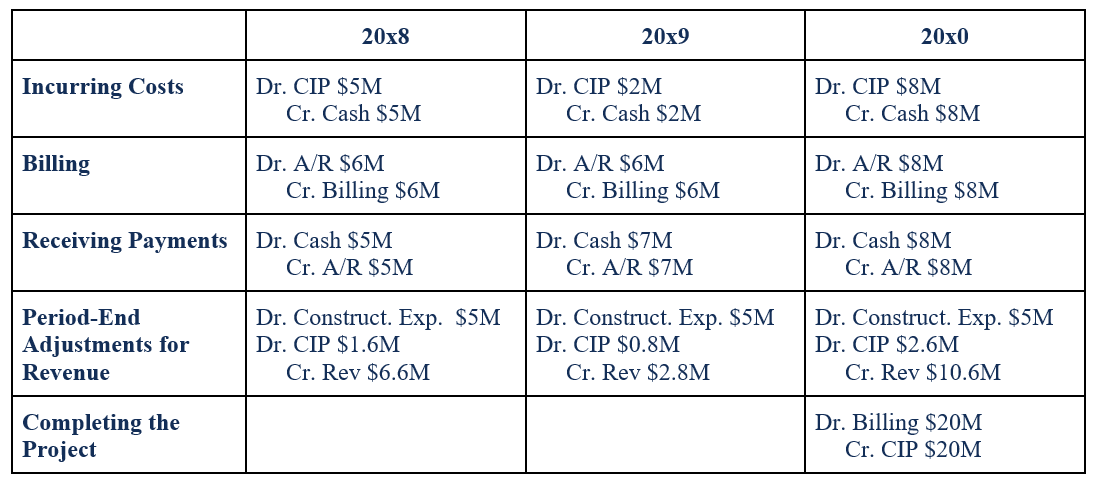CFI is the official provider of the Financial Modeling and Valuation Analyst (FMVA)™ certification program, designed to transform anyone into a world-class financial analyst.

To keep learning and developing your knowledge of financial analysis, we highly recommend the additional CFI resources below:

• Evaluation Plan
• Project Budget Template
• Project Finance
• Raid Log